# Reinhardt domain

(diff) ← Older revision | Latest revision (diff) | Newer revision → (diff)

multiple-circled domain

A domainin the complex space,, with centre at a point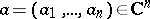, with the following property: Together with any point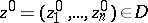, the domain also contains the setA Reinhardt domainwith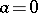is invariant under the transformations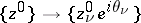,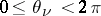,. The Reinhardt domains constitute a subclass of the Hartogs domains (cf. Hartogs domain) and a subclass of the circular domains, which are defined by the following condition: Together with any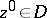, the domain contains the set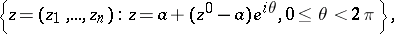i.e. all points of the circle with centreand radius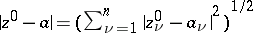that lie on the complex line throughand.

A Reinhardt domainis called a complete Reinhardt domain if together with any pointit also contains the polydiscA complete Reinhardt domain is star-like with respect to its centre(cf. Star-like domain).

Examples of complete Reinhardt domains are balls and polydiscs in. A circular domainis called a complete circular domain if together with any pontit also contains the entire disc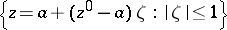.

A Reinhardt domainis called logarithmically convex if the imageof the set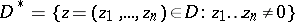under the mapping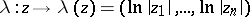is a convex set in the real space. An important property of logarithmically-convex Reinhardt domains is the following: Every such domain inis the interior of the set of points of absolute convergence (i.e. the domain of convergence) of some power series in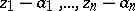, and conversely: The domain of convergence of any power series in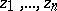is a logarithmically-convex Reinhardt domain with centre.

How to Cite This Entry:
Reinhardt domain. Encyclopedia of Mathematics. URL: http://encyclopediaofmath.org/index.php?title=Reinhardt_domain&oldid=16774
This article was adapted from an original article by E.D. Solomentsev (originator), which appeared in Encyclopedia of Mathematics - ISBN 1402006098. See original article